Courses

# Test: Thin & Thick Walled Pressure Vessels - 2

## 10 Questions MCQ Test Topicwise Question Bank for Mechanical Engineering | Test: Thin & Thick Walled Pressure Vessels - 2

Description
This mock test of Test: Thin & Thick Walled Pressure Vessels - 2 for Mechanical Engineering helps you for every Mechanical Engineering entrance exam. This contains 10 Multiple Choice Questions for Mechanical Engineering Test: Thin & Thick Walled Pressure Vessels - 2 (mcq) to study with solutions a complete question bank. The solved questions answers in this Test: Thin & Thick Walled Pressure Vessels - 2 quiz give you a good mix of easy questions and tough questions. Mechanical Engineering students definitely take this Test: Thin & Thick Walled Pressure Vessels - 2 exercise for a better result in the exam. You can find other Test: Thin & Thick Walled Pressure Vessels - 2 extra questions, long questions & short questions for Mechanical Engineering on EduRev as well by searching above.
QUESTION: 1

### What is the thickness of the shell of a bomb calorimeter of spherical form of 10 cm inside diameter if the working stress is σMPa and the internal pressure is σ/2 MPa? [Take 21/3 = 1.26]

Solution: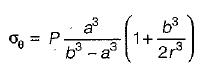at r = a, σθ is maximum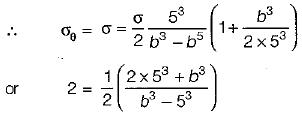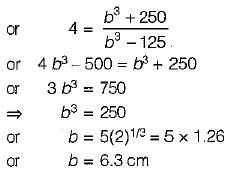Thickness of bomb shell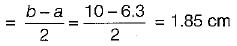QUESTION: 2

Solution:
QUESTION: 3

### Hoop stress in a thin cylinder of diameter dand thickness t subjected to pressure p will be

Solution:

Due to fluid pressure p in the cylinder bursting force = p x d x L
This is resisted by thickness of cylinder Resisting force = σh(2t x L).
For equilibrium
Resisting force = Bursting force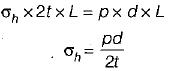where σh = hoop stress
p = internal fluid pressure
d = Diameter of cylinder
t = Thickness of cylinder

QUESTION: 4

Longitudinal stress in a thin cylinder of diameter d and thickness t subjected to pressure p will be

Solution: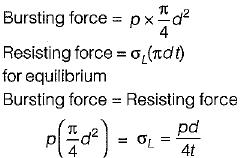QUESTION: 5

A thin cylindrical shell of internal diameter d and thickness ‘t’ is subjected to internal pressure ‘p’. The change in diameter is given by

Solution: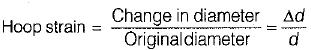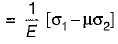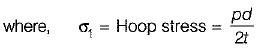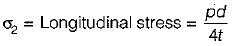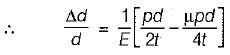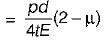∴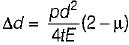QUESTION: 6

In above case the longitudinal strain is

Solution: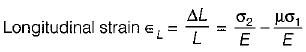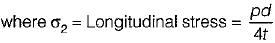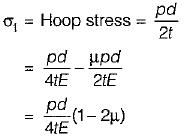QUESTION: 7

A thin cylindrical shell of diameter d, length L and thickness t is subjected to an internal pressure p. What is the ratio of longitudinal strain to hoop strain in terms of Poisson’s ratio (1/m)?

Solution: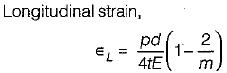Hoop strain,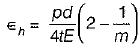∴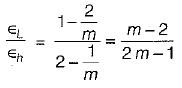QUESTION: 8

The volumetric strain in case of a thin cylindrical shell of diameter d, thickness t, subjected to internal pressure p is

Solution: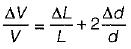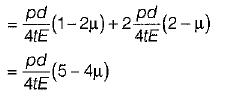QUESTION: 9

The design of thin cylindrical shells is based on

Solution:

Since hoop stress (σh) > Longitudinal stress (σL). Hence to have safe design, hoop stress is considered.

QUESTION: 10

The commonly used technique of strengthening thin pressure vessels is

Solution:

The commonly used technique of strengthening thin pressure vessels is wire winding. The wire is wound under tension, thus putting the walls of the cylinder under compression. This way, the cylinder is pre-stressed.